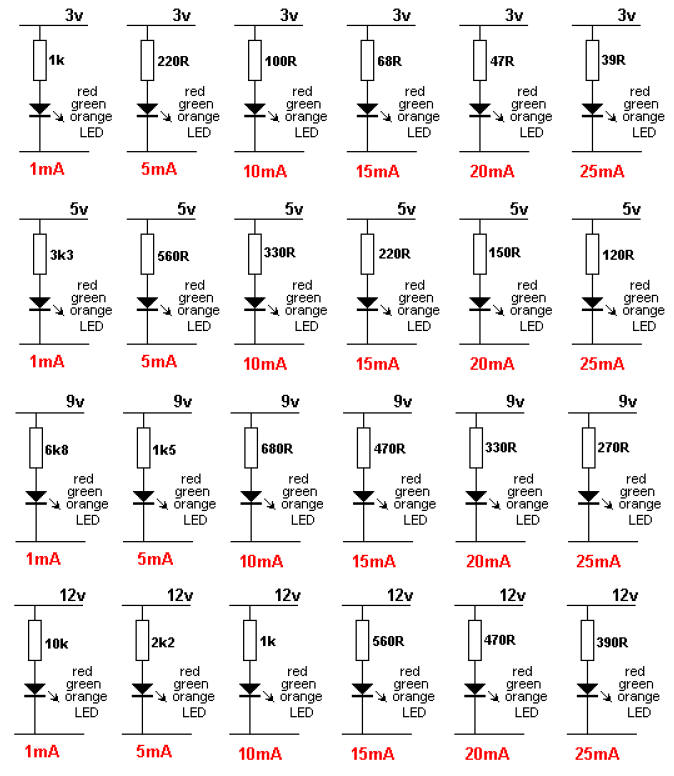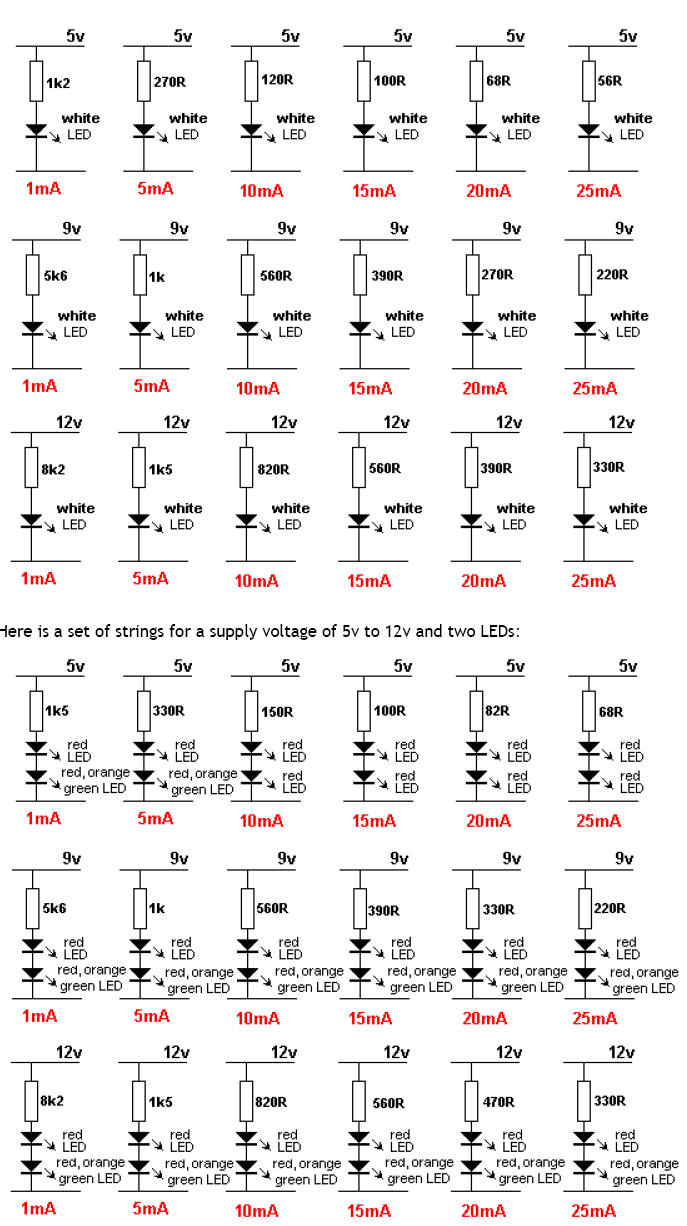The resistor
Electronic

The value of the current limiting resistor can be worked out by Ohms Law. Here are the 3 steps:

1. Add up the voltages of all the LEDs in a string. e.g: 2.1v + 2.3v + 2.3v + 1.7v = 8.4v

2. Subtract the LED voltages from the supply voltage. e.g: 12v - 8.4v = 3.6v

3. Divide the 3.6v (or your voltage) by the current through the string.

for 25mA: 3.6/.025 =144 ohms for 20mA: 3.6/.02 = 180 ohms

for 15mA: 3.6/.015 = 250 ohms for 10mA: 3.6/.01 = 360 ohms

This is the value of the current-limiting resistor.

Here is a set of strings for a supply voltage of 3v to 12v and a single LED:Here is a set of strings for a supply voltage of 5v to 12v and a white LED:Recherche personnalisée